# Civil Engineering - Theory of Structures

### Exercise :: Theory of Structures - Section 3

31.

Pick up the correct statement from the following:

 A. The structural member subjected to compression and whose dimensions are small as compared to its length, is called a stmt B. The vertical compression members, are generally known as columns or stanchions C. Deflection in lateral direction of a long column, is generally known as buckling D. All the above.

Explanation:

No answer description available for this question. Let us discuss.

32.

A short column (30 cm x 20 cm) carries a load P1 at 4 cm on one side and another load P2 at 8 cm on the other side along a principal section parallel to longer dimension. If the extreme intensity on either side is same, the ratio of P1 to P2 will be

 A.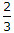B.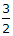C.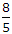D.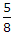Explanation:

No answer description available for this question. Let us discuss.

33.

 A. magnitude B. direction C. point of application D. all the above.

Explanation:

No answer description available for this question. Let us discuss.

34.

Slenderness ratio of a long column, is

 A. area of cross-section divided by radius of gyration B. area of cross-section divided by least radius of gyration C. radius of gyration divided by area of cross-section D. length of column divided by least radius of gyration.

Explanation:

No answer description available for this question. Let us discuss.

35.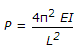is the equation of Euler's crippling load if

 A. both at the ends are fixed B. both the ends are hinged C. one end is fixed and other end is free D. one end is fixed and other end is hinged.